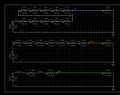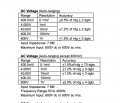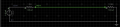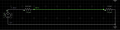# Build a high voltage probe to calibrate a 1.5Kv oscilloscope CRT

#### gkmaia

Joined Dec 22, 2018
34
I want to build a high voltage probe to calibrate my 1.5Kv oscilloscope CRT supply voltage.

Will just use this probe once to calibrate the scope so I don’t want to spend \$200 on a probe and I mind the safety risks that it may involve.

Have seen several project examples. Some use several small resistors in series with capacitors in between. Others just resistors. Other just one HV resistor.

But before building anything I should consider what fits my multimeter DC Input Impedance which is 7.8MOhm, right?

So what I need is basically a voltage divider capable of reducing the voltage 10 times. A 70MOhm probe should achieve the 10x attenuation and bring 1.5kv down to 150v if I am not wrong.

I am planning to use one 50MOhm and one 20MOhm in series. Both High voltage resistors, rated at 1.5Kv and 1/2W. Not planning to use any capacitors as well.

Frequency wise will I have any issues considering just this one time CRT calibration?

Is it worth a shot?

#### Hymie

Joined Mar 30, 2018
1,135
Normally the input impedance of a digital multimeter (when measuring voltage) is 10MΩ, I don’t know where your figure of 7.8MΩ is from.

But rather than rely on the meter impedance to achieve the voltage division, I would advise forming a complete resistor divider chain, measuring across the resistor at the low end of the chain.

To achieve a division of 10 you could connect ten resistors of 100kΩ in series. The load on the 1.5kV would be less than 2.5W, with 150V across each 100kΩ resistor.

Ten 100kΩ ¼ watt resistors will work out cheaper than the cost of the 70MΩ resistor.

Joined Jan 15, 2015
7,193
You may want to give this a read. The link includes a diagram of the Fluke 80K-40 HV probe. Consider the insulation properties of your resistors and material you make the probe with. Note where the link covers the input impedance of the voltmeter used which as Hymie mentions is typically 10 Meg Ohm. Consider that when choosing your resistor values. The voltage should be DC so frequency should not be an issue.

Ron

#### gkmaia

Joined Dec 22, 2018
34
Normally the input impedance of a digital multimeter (when measuring voltage) is 10MΩ, I don’t know where your figure of 7.8MΩ is from.
From its service manual. See attached.

There are few scenarios. Your 100K scenario under a 10Mohm meter would result on 1v drop per resistor. The final output would be 1.36Kv.

Considering my awkward meter impedance, the best approach would be 7 X 10MOHm in series. That would load each resistor with a 200V drop. Is that a fair assumption?Last edited:

#### gkmaia

Joined Dec 22, 2018
34
You may want to give this a read. The link includes a diagram of the Fluke 80K-40 HV probe. Consider the insulation properties of your resistors and material you make the probe with. Note where the link covers the input impedance of the voltmeter used which as Hymie mentions is typically 10 Meg Ohm. Consider that when choosing your resistor values. The voltage should be DC so frequency should not be an issue.

Ron
Thanks for that. That helps understanding it better. Fluke does a 1000:1 attenuation by adding 1GOhm to a 10Mohm meter. That would bring the 1.5Kv down to 14.9 volts. But if it were 990Mohm then it would go perfectly down to 15v.

I guess the reason why so many resistors in series has not much to do with power but more to reduce the voltage drop load on each resistor. Is that correct?#### crutschow

Joined Mar 14, 2008
32,088
You need to consider the load on the CRT supply.
You want the probe to draw only a small fraction of the normal CRT HV current draw (which I assume is quite small).

#### DickCappels

Joined Aug 21, 2008
9,877
It looks like a simple voltage divider. The important features are (as crutschow has pointed out) to have a high enough resistance in your divider and to make sure that the voltage across all resistors are not beyond the voltage handling ability of those resistor.

#### crutschow

Joined Mar 14, 2008
32,088
I saw one reference that stated a typical oscilloscope CRT anode current was 250μA, so you can use that as a starting point.
You would want the probe to draw much less current than that (perhaps only a percent or so).

#### BR-549

Joined Sep 22, 2013
4,928
One way to get around the high voltage concern for the resistors..........series enough resistors, so that only about 100 volts is across each one.

And as crutschow says.....only allow a very small current.

Never put meter in series with high voltage.

https://www.repairfaq.org/sam/hvprobe.htm#shvbas